Next: The Gravimagnetic Parameter Up: ``Ecology'' of Magnetic Rotators Previous: Nomenclature

## A Universal Diagram for Gravimagnetic Rotators

ĀĀ

The classificationĀ given above was based on relations between the characteristic radii, i.e. quantities which cannot be observed directly. This drawback can be removed if we note that the light cylinder radius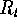, Schwartzman radiusĀ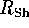and corotation radiusĀ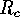are functions of the well-observed quantity, rotational period of the magnetorĀ p. Hence, the above classification can be reformulated in the form of inequalities for the rotational period of a magnetic rotator.

One can introduce two critical periods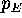and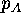such that their relationship with period p of a magnetic rotator specifies the rotator's type:The values ofandcan be determined from TableĀ2 which defines the basic nomenclature, and are functions of the parameters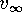,,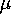and. The parameters p andcharacterize the electromagnetic interaction, whiledescribes the gravitational interaction. Instead of, we introduce the potential accretion luminosity L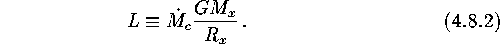The physical sense of the potential luminosity is quite clear: the accreting star would be observed to have this luminosity if the matter formally falling on the gravitational capture cross-section were to reach its surface.

Approximate expressions for critical periods (Lipunov 1992) are

ĀHere a new critical periodwas introduced from the condition: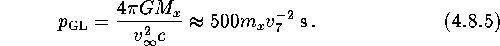Treating the rotator's magnetic dipole momentandas parameters, we find that an overwhelming majority of the magnetor's stages can be shown on a ``'' diagram (Lipunov 1982a). The quantity L also proves to be convenient because it can be observed directly at the accretion stage.

Mike E. Prokhorov
Sat Feb 22 18:38:13 MSK 1997